Tutorial 13 – Generators in Python

Welcome to the thirteenth tutorial of Selenium-Python series! In this tutorial, we will study about Generators in python.

What you will Learn:
• Generators
• Return versus Yield
• Generator versus Function
• next()
• yield
• Generator example
• Conclusion

Generators
Let us quickly recall the ‘for’ loop that iterates over a list, see below. The ‘for’ loop iterates over each member of the list & prints it. We have already covered lists earlier in data structures tutorial.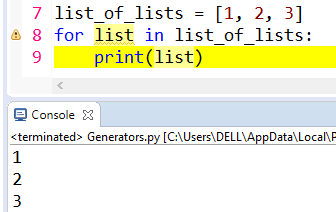Figure 1

To understand generators, let us take an example. We create a function ‘squareNumbers’ and pass the ‘nums’ argument. We will use this function to print the square of the numbers. See below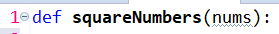Figure 2

Let us create an empty list over here, see below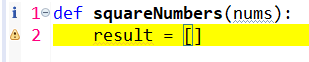Figure 3

Let us now write a ‘for’ loop, see lines 3 & 4 below. The append() method adds an item to the end of the list. So the append() method modifies the original list.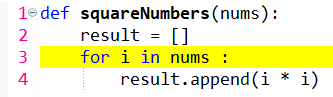Figure 4

Next we will return the return, see below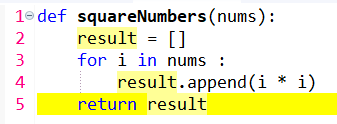Figure 5

To call the ‘squareNumbers’ function, we write line number 7 and pass a list [1,2,3], see belowFigure 6

We next print the my_nums result, see below. When we run the program, we see that the square of the numbers gets printed in console.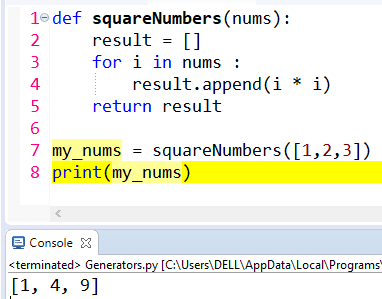Figure 7

But the question arises is, why do we have to create an empty list (line 2 above)? Why do we have to create this complicated code? To simplify the things, we use generators.
Look at the below code. The line numbers 2 and 5 seen in the above program have been removed in the below program. Also, instead of append method, we are using ‘yield’.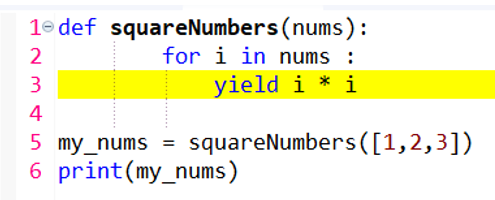Figure 8

When you run the program, we get an o/p “<generator object squareNumbers at 0x007D6F30>”, see below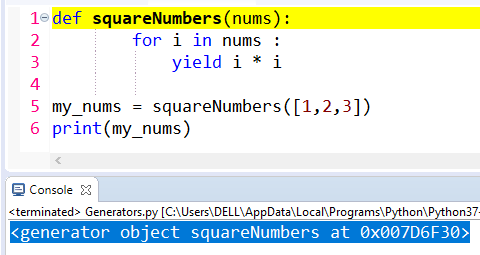Figure 9

So what exactly these generators are? These generators are a simple way of creating the iterators, like, you see over here. It is a function that returns an ‘object’. If you again look at the console o/p, it returned us the generator object at a memory location “<generator object squareNumbers at 0x007D6F30>”

Return versus Yield
Both, ‘yield’ & ‘return’ will return us some value from the function. However there are few differences:
-The return is a final statement of a function. It provides a way to send some value back. While returning, its local stack gets flushed. And any new call will begin execution from the very first statement.
-The yield preserves the state between subsequent function calls. It resumes execution from the point where it gave back the control to the caller viz right after the last yield statement.
So in essence, the difference is that, the ‘return’ terminates the function entirely but the ‘yield’ statement first gives us the object & after that it continues with successive calls. Thus ‘yield’ does not terminate the function. Also, yield will iterate over one value at a time.
Coming back, see line number 6 in the below figure. We have used ‘next’ function. The next() function returns the next item in an iterator. The syntax of next() is: next(iterator).
The next() function parameter 'iterator' retrieves next item from the iterator. Here, our iterator is ‘my_nums’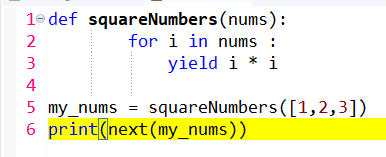Figure 10

So this will print us the square of the number viz 1 (square of 1)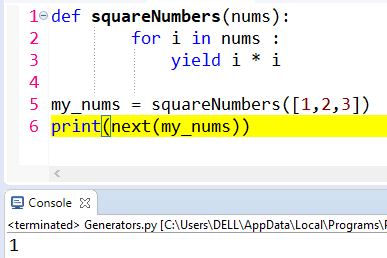Figure 11

To print the square of other numbers in the list, simply copy/paste the same print statement as seen below. We see 1, 4 and 9 in the o/p. So this is how the generator works. So it is very simple code. Rather than storing the values in a list, it simply gives us the value one at a time. The generator does NOT store the values in the memory & than gives us the o/p. It will simply give us the o/p one value at a time & memory utilization is reduced.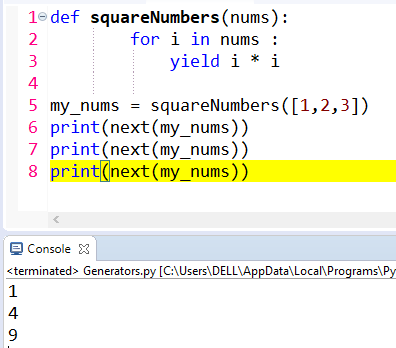Figure 12

If we copy/paste one more additional print statement, see below, ‘StopIteration’ would be printed in the o/p, since we have exhausted the list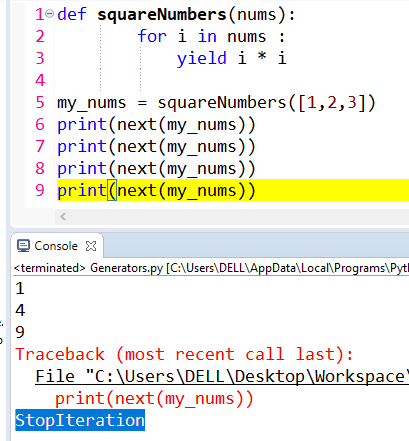Figure 13

To avoid ‘StopIteration’, we can use a ‘for’ loop. So let us comment the print statements (lines 6,7 and 8 below). You can also remove them from program if you want.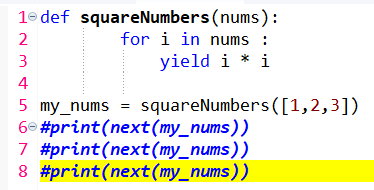Figure 14

So look at the ‘for’ loop below that will print the square of all the numbers. Now we do not get the ‘StopIteration’ error.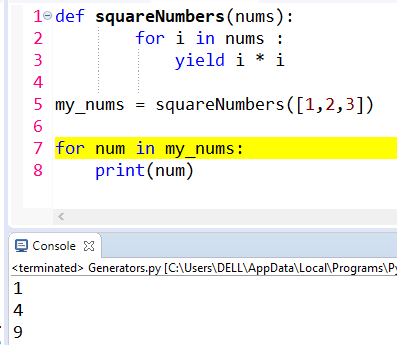Figure 15

If we add few more numbers in the list & run the program, we get desired o/p.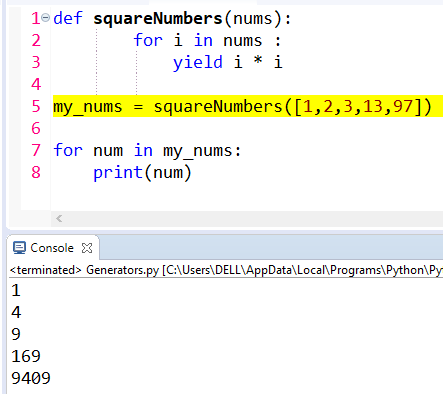Figure 16

Generator versus Function
The difference between a generator and a regular function:
-A generator uses the yield statement to send a value back to the caller whereas a function does it using the return.
-The generator function can have one or more than one yield call.
-The yield call pauses the execution and returns an iterator, whereas the return statement is the last one to be executed.
-The next() method call triggers the execution of the generator function.

next() function example
Below is an another example of next() function. In line number 3, we are converting list to iterator. Rest is self-explanatory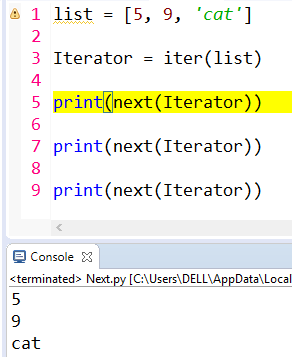Figure 17

yield example
Below is another yield example. gen_func() yields 1 in the first call, 2 in the second, and 3 during the third.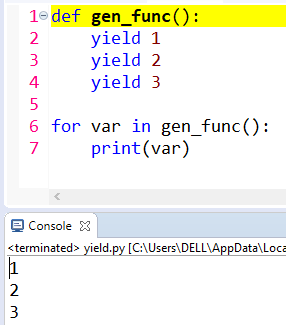Figure 18

Generator example
Below is another generator example that is self-explanatory.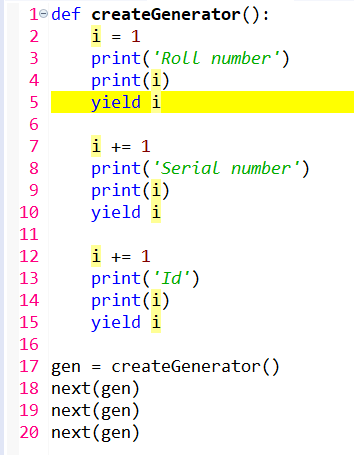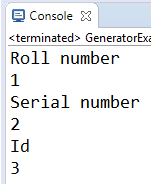Figure 19

Conclusion
In this tutorial we studied about generators in python. A generator is a function that returns an object which we can iterate over one value at a time. Thus generators allow programmers to make an iterator in a fast, easy, and clean way. Thank you for reading!